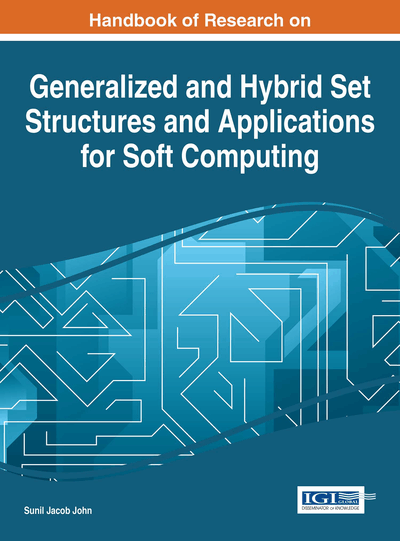# Generalized Fuzzy Closed Sets in Smooth Bitopological Spaces

Osama A. El-Tantawy (Zagazig University, Egypt), Sobhy A. El-Sheikh (Ain Shams University, Egypt) and Rasha N. Majeed (Ain Shams University, Egypt & Baghdad University, Iraq)
Copyright: © 2016 |Pages: 38
DOI: 10.4018/978-1-4666-9798-0.ch020
OnDemand PDF Download:
Available
\$37.50
No Current Special Offers

## Abstract

This chapter is devoted to the study of r-generalized fuzzy closed sets (briefly, gfc sets) in smooth bitopological spaces (briefly, smooth bts) in view definition of Šostak (1985). The chapter is divided into seven sections. The aim of Sections 1-2 is to introduce the fundamental concepts related to the work. In Section 3, the concept of r-(ti,tj)-gfc sets in the smooth bts's is introduce and investigate some notions of these sets, generalized fuzzy closure operator induced from these sets. In Section 4, (i,j)-GF-continuous (respectively, irresolute) mappings are introduced. In Section 5, the supra smooth topology which generated from a smooth bts is used to introduce and study the notion of r-t12-gfc sets for smooth bts's and supra generalized fuzzy closure operator . The present notion of gfc sets and the notion which introduced in section 3, are independent. In Section 6, two types of generalized supra fuzzy closure operators introduced by using two different approaches. Finally, Section 7 introduces and studies different types of fuzzy continuity which are related to closure.
Chapter Preview
Top

## 1. Introduction

Šostak (1985) introduced the fundamental concept of a ‘fuzzy topological structure’, as an extension of both crisp topology and Chang’s fuzzy topology Chang (1968) indicating that not only the object were fuzzified, but also the axiomatics. Subsequently, Badard (1986) introduced the concept of ‘smooth topological space’. Chattopadhyay et al. (1992) and Chattopadhyay and Samanta (1993) re-introduced the same concept, calling it ‘gradation of openess’. Ramadan (1992) and his colleagues introduced a similar definition, namely, smooth topological space for lattice L=[0,1]. Following Ramadan, several authors have re-introduced and further studied smooth topological space (cf. Chattopadhyay et al. (1992), Chattopadhyay and Samanta (1993), Chen (2011), El Gayyar et al. (1994), Šostak (1996)). Thus, the terms ‘fuzzy topology’, in Šostak’s sense, ‘gradation of openness’ and ‘smooth topology’ are essentially referring to the same concept. In This chapter, the term smooth topology is adopted. Lee et al. (2001) introduced the concept of smooth bitopological space as a generalization of smooth topological space and Kandil’s fuzzy bitopological spaces Kandil (1989).

Levine (1970) introduced the concept of generalized closed sets of a topological space and a class of topological spaces calledspaces, and many authors investigated their properties (cf. (Dunham (1977), Dunham and Levine (1980), Dunham (1982)). Subsequently, Fukutake (1986) introduced the concept of generalized closed sets in bitopological spaces. Balasubramanian and Sundaram (1997) introduced the concept of generalized fuzzy closed sets within Chang’s fuzzy topology, as an extension of the generalized closed sets of Levine. Kim and Ko (2003) defined r-generalized fuzzy closed sets in smooth topological spaces.

The so-called supra topology was established by Mashhour et al. (1983) (recall that a supra topology on a set X is a collection of subsets of X, which is closed under arbitrary unions). Abd El-Monsef and Ramadan (1987) introduced the concept of supra fuzzy topology, followed by Ghanim et al. (2000) who introduced the supra fuzzy topology in Šostak sense. Abbas (2002) generated the supra fuzzy topology (X12) from fuzzy bitopological space (X12) in Šostak sense as an extension of supra fuzzy topology due to Kandil et al. (1995).

This chapter is devoted to the study of r-generalized fuzzy closed sets in smooth bitopological spaces in view of the definition of Šostak (1985), and constructs some types of fuzzy closure operators, smooth topologies, supra smooth topologies and mappings. The research work carried out and reported in this chapter is divided into seven sections. The aim of Sections 1-2 is to introduce the fundamental concepts related to this chapter.

In Section 3, the concept of r-(τi,j) -generalized fuzzy closed sets in the smooth bitopological spaces have been introduced and investigate some notions of these sets. By using r-(τi,𝜏j)-gfc sets, a new fuzzy closure operator referred to asis define which generates a new smooth topology,(see Theorem 3.2).

## Complete Chapter List

Search this Book:
Reset